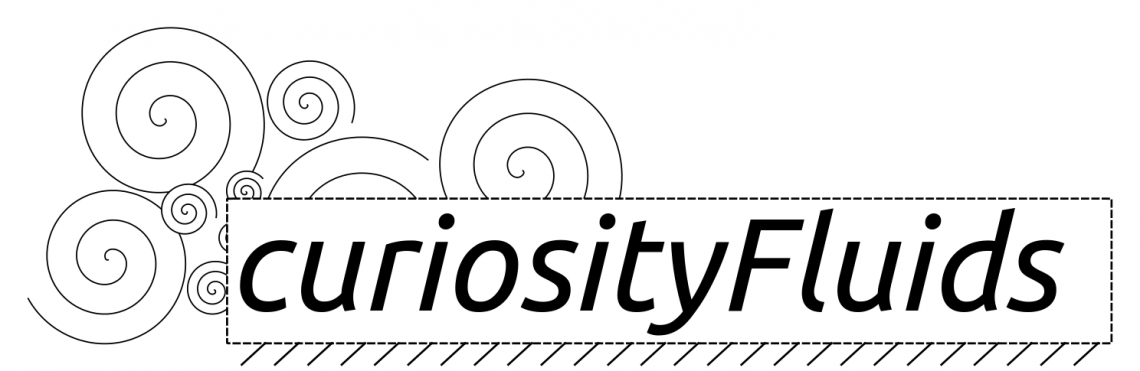Skip to content# Couette Flow (Moving Wall)

In this post I am going to go through the solution to the moving wall Couette flow problem.

An illustration of the problem is given below:

In this problem, the fluid between two parallel plates is being driven by the motion of the top plate. Here, we assume that the flow is axial (v=w=0), incompressible (ρ=constant), fully developed (u only a function of y). We also assume that gravity can be neglected and that no pressure gradient is present (dp/dx=0).$\rho\left(u\frac{\partial u}{\partial x}+ v \frac{\partial u}{\partial y}\right) = \frac{\partial p}{\partial x} + \mu\left( \frac{\partial^2 u}{\partial y^2}+\frac{\partial^2 u}{\partial y^2}\right)$

By applying our assumptions listed above, you should be able to see that the equation simply becomes:$\frac{d^2 u}{dy^2}=0$

To solve the problem we must integrate this equation and solve using the boundary conditions defined by the problem. The integration results in:$u=Ay+B$

Our boundary conditions come from the problem and our super smart knowledge of the no-slip condition ;). Ie.

@$y=h$,$u=V$

@$y=0$,$u=0$

After subbing in we get two equations, with two unknowns (the integration constants)$V=Ah+B$$0=A(0)+B$

Therefore we can see that B=0 and A=V/h. This leads to the final solution of this plane Couette flow problem:$u=\frac{Vy}{h}$

Now we have shown that the velocity profile in this case is the linear profile above. We can also calculate the shear stress:$\tau=\mu \frac{du}{dy} = \mu \frac{V}{h}$Categories

Basic Fluids

Tags1.jainil soni says:
2.ishaaq123 says: